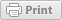# Equation to Estimate Sample Size Required for QTL Detection

Plant Breeding and Genomics May 16, 2012Authors:

David M. Francis, The Ohio State University; Heather L. Merk, The Ohio State University

This page provides an equation to estimate the required sample size to detect quantitative trait loci (QTLs) of varying effect in F2 and BC1 populations.

## Introduction

Population size, structure, and the number of molecular markers genotyped can have significant impacts on quantitative trait locus (QTL) detection. This page focuses on the effect population size can have on QTL detection. Prior knowledge of theories regarding the genetics controlling the trait can help guide breeders as to the number of plants required to detect QTLs of various sizes using the following equation. Solving the equation below can help breeders optimize resources while still gaining the desired outcome.

## Equation to Calculate Minimum Sample Size

Using units of phenotypic standard deviations, sample sizes can be estimated using the following equations:

For an F2 population,

NF2 = [1 - r2F2 / r2F2] x {[z(1 - (α / 2)) / (1 - r2F2)1/2] + z(1 - β)}2 x [1 + (k2 / 2)]

For a BC1 population,

NBC1 = [1- r2BC1 / r2BC1 ] x {[z (1 - (α / 2)) /(1 - r2BC1)1/2 ] + z(1 – β)}2 x [1 + (k2 / 2)]

In these equations,

• r2 is the fraction of phenotypic variance explained by the QTL. Note that r2 is valid only for simple models. Mean Squares provide an alternative method to estimate fraction of phenotypic variation when models have replication and location.
• k is the dominance coefficient (k = 0 for completely additive trait, k = 1 for completely dominant trait and k = -1 for completely recessive trait). This equation assumes that the marker and QTL are coincidental.
• α is the type I error (the probability of incorrectly identifying an association that does not, in fact, exist)
• β is the type II error (the probability of failing to identify a true association)
• From normal distribution tables:
• z(1-(α/2)) = 1.96 at α = 0.05
• z(1–β) = 1.28 at ß = 0.10

For example, in an F2 population, the estimated sample size required to detect a dominant QTL that is responsible for 30% of the phenotypic variation is 46.

NF2 = [(1 - 0.30) / 0.30] x {[1.96 / (1 - 0.30)1/2] + 1.28}2 x [1 + (12 / 2)] = 46

## Funding Statement

Development of this lesson was supported in part by the National Institute of Food and Agriculture (NIFA) Solanaceae Coordinated Agricultural Project, agreement 2009-85606-05673, administered by Michigan State University. Any opinions, findings, conclusions, or recommendations expressed in this publication are those of the author(s) and do not necessarily reflect the view of the United States Department of Agriculture.

PBGworks 862

### Welcome

This is where you can find research-based information from America's land-grant universities enabled by eXtension.orgThis work is supported by the USDA National Institute of Food and Agriculture, New Technologies for Ag Extension project.